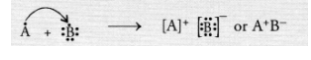# Write the formula of the compound formed

Question:

Write the formula of the compound formed when the element A (atomic number 19) combines with the element B (atomic number 17). Draw its

electronic dot structure. ^What is the nature of the bond formed ? (CBSE 2013)

Solution:

Electronic configuration of element A (Z = 19) is 2, 8, 8, 1

Electronic configuration of element B(Z = 17) is 2, 8, 7.

The element A has one valence electron while the element B has seven electrons in its valence shell. One electron gets transferred from the atom

of element A to the atom of element B. As a result of electron transfer, an ionic bond is formed.